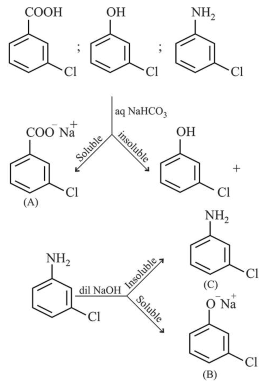# A solution of m-chloroaniline,Question:

A solution of $m$-chloroaniline, $m$-chlorophenol and $m$-chlorobenzoic acid in ethyl acetate was extracted initially with a saturated solution of $\mathrm{NaHCO}_{3}$ to give fraction $A$. The left over organic phase was extracted with dilute $\mathrm{NaOH}$ solution to give fraction $B$. The final organic layer was labelled as fraction $C$. Fractions $A, B$ and $C$, contain respectively:

1. $m$-chlorobenzoic acid, $m$-chloroaniline and $m$-chlorophenol

2. $m$-chlorobenzoic acid, $m$-chlorophenol and $m$-chloroaniline

3. $m$-chlorophenol, $m$-chlorobenzoic acid and $m$-chloroaniline

4. $m$-chloroaniline, $m$-chlorobenzoic acid and $m$-chlorophenol

Correct Option: , 2

Solution: